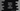# What is __init__ in Python## init in Python :

If you know how to create python class and objects, you must have seen init method before. For the first comers, this looks different than other methods and classes. In this blog post, I will explain to you what is init and when/how to use it with different examples. Go through the tutorial and drop a comment below if you have any queries.

### init as a constructor :

Constructors are methods that gets called first when we create one object. If you know any other object oriented programming language like C++, Java, JavaScript, you might be familiar with it. In python, init method is same as like constructors. It gets called first.

So, we can use this method to do all starting tasks like initializing the instance variables, initialize other class objects etc. This method runs as soon as the object is created. For example, let’s consider the below example program :

``````class Student:
def __init__(self,name):
self.name = name
student = Student('Alex')
print('Student name : {}'.format(student.name))``````

If you run it, it will print the below output :

``Student name : Alex``

Here, we are creating one instance of the class Student. String ‘Alex’ is passed as the argument to Student class. The init method is called first once the object is created or it is initialized. ‘self’ is used to represent the current instance. Using it, we are binding one attribute with the argument. The last line is printing the ‘name’ of the object ‘student’.

### init in superclass :

We can invoke init in the super class before executing on the child class using super() method. For example :

``````class Car:
def __init__(self, color):
print('__init__ in Car')
self.color = color

class Audi(Car):
def __init__(self, name, color):
super().__init__(color)
print('__init__ in Audi')
self.name = name

audi = Audi('XX', 'red')

print('name : {}, color : {}'.format(audi.name, audi.color))``````

Here, Car is the super class of Audi and we are calling this class when the init() of Audi is called. We are using super() keyword to call this classs or super class. It will invoke the init() method of the super class first and then the sub class. Below is output of this program :

``````__init__ in Car
__init__ in Audi
name : XX, color : red``````